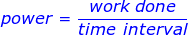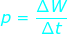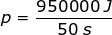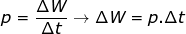# Power Formula

Power Formula

Power is a rate at which work is done, or energy is used. It is equal to the amount of work done divided by the time it takes to do the work. The unit of power is the Watt (W), which is equal to a Joule per second (J/s).P = power (W, or J/s)

∆W = the work done, or energy used (J)

∆t = the time taken to do the work (s)

Power Formula Questions:

1) Raising an elevator from the bottom to the top of a building requires 950000 J of work. The elevator takes 50 seconds to reach the top. How much power was used to raise the elevator?

Answer: The work done to raise the elevator was 950000 Joules, and the time interval was 50 seconds. The power can be found using the formula:P = 19000 W

P = 19 kW

The power required to raise the elevator was 19000 Watts, which is equal to 19 kW.

2) How much energy is used by a 60-Watt light bulb that has been left on for eight hours?

Answer: The power of the light bulb is given, as well as a time interval. The time is 8 hours, or (8 hours)(60 minutes/hour)(60 seconds/minute) = 28800 s. The energy used can be found by rearranging the equation:∆W = P∙∆t

∆W = (60 W)∙(28800 s)

∆W = 1728000 J

∆W = 1728 kJ

The energy expended by the 60-Watt light bulb in 8 hours was 1728000 J, or 1728 kJ.